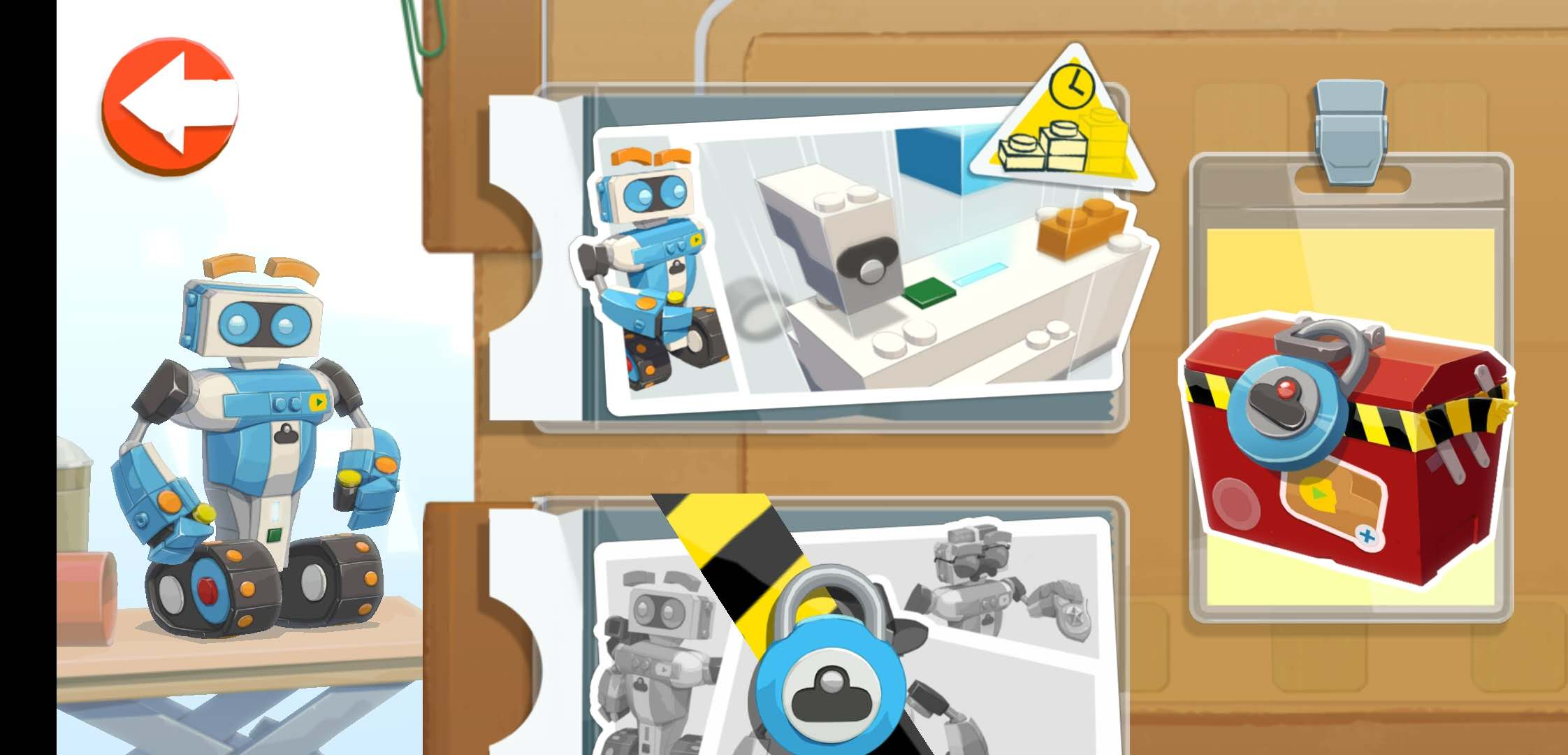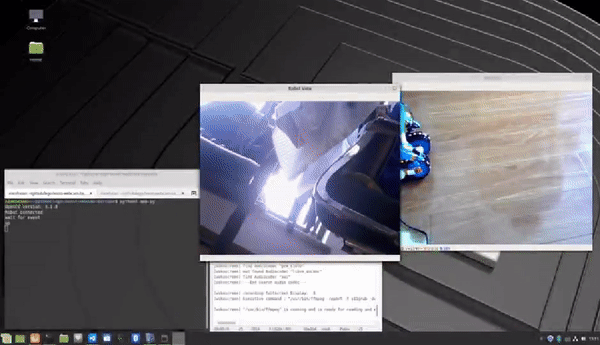# Making a Barcode Scan Robot with Lego Boost and Webcam

If you want to quickly create a walking robot, you can use the Lego Boost. In this post, I will share how to use a webcam, Lego Boost, and Dynamsoft Barcode Reader SDK to make a robot for scanning barcodes. The programming language used in this article is Python.

## How to Build the Robot with Lego Boost

To build the robot, install the Boost app and follow the relevant tutorials.## Python Programming Requirements

``````    pip install pygatt
pip install gatt
pip install gattlib
pip install bluepy
``````
• Install pylgbst to interact with Lego Boost Move Hub:
``````    pip install https://github.com/undera/pylgbst/archive/1.0.tar.gz
``````
• Install OpenCV Python:
``````    pip install opencv-python
# or
pip3 install opencv-python
``````

## How to Control Lego Boost Robot to Scan Barcodes

Create a camera view window using OpenCV:

``````    vc = cv2.VideoCapture(0)
vc.set(3, 640) #set width
vc.set(4, 480) #set height

if vc.isOpened():  # try to get the first frame
else:
return

windowName = "Robot View"

try:
while True:
cv2.imshow(windowName, frame)

``````

Due to GIL (Python Global Interpreter Lock), we need to run Bluetooth connection code and barcode decoding algorithm in another process rather than thread. All data, including key events, webcam frames, and barcode results, are transferred via Queue:

``````    num = Value('i', 1)
result _queue = Queue(1)
key _queue = Queue(1)
frame _queue = Queue(1)
cond = Condition()
dbr _proc = Process(target=dbr _run, args=(
frame _queue, key _queue, cond, num, result _queue))
dbr _proc.start()

``````

Here are the key definitions:

• a: left
• d: right
• w: up
• s: down
• q: terminate the app
• c: capture images and scan barcodes

The code for controlling the Lego Boost Robot is pretty simple. I use Gatt Backend:

``````conn GattConnection()
try:
conn.connect()
hub = MoveHub(conn)
print('Robot connected')
speed = 0.5

if key == ord('a'):
# left
hub.motor _AB.angled(90, speed * -1, speed)
elif key == ord('d'):
# right
hub.motor _AB.angled(90, speed, speed * -1)
elif key == ord('w'):
# up
hub.motor _AB.start _speed(speed)
elif key == ord('s'):
# down
hub.motor _AB.start _speed(speed * -1)
elif key == ord('p'):
hub.motor _AB.stop()
finally:
conn.disconnect()
``````

Read the QR code and put the results into the queue:

``````dbr.initLicense('DLS2eyJoYW5kc2hha2VDb2RlIjoiMjAwMDAxLTE2NDk4Mjk3OTI2MzUiLCJvcmdhbml6YXRpb25JRCI6IjIwMDAwMSIsInNlc3Npb25QYXNzd29yZCI6IndTcGR6Vm05WDJrcEQ5YUoifQ==')

if key == ord('c'):
inputframe = frame _queue.get()
results = dbr.decodeBuffer(inputframe, 0x4000000)
if (len(results) > 0):
for result in results:
print("Type: " + result)
print("Value: " + result + "\\n")

result _queue.put(Result(inputframe, results))
``````

Draw the barcode position and decoded text result using OpenCV:

``````                ret = result _queue.get _nowait()
results = ret.results
image = ret.image

thickness = 2
color = (0,255,0)
for result in results:
print("barcode format: " + result)
print("barcode value: " + result)
x1 = result
y1 = result
x2 = result
y2 = result
x3 = result
y3 = result
x4 = result
y4 = result

cv2.line(image, (x1, y1), (x2, y2), color, thickness)
cv2.line(image, (x2, y2), (x3, y3), color, thickness)
cv2.line(image, (x3, y3), (x4, y4), color, thickness)
cv2.line(image, (x4, y4), (x1, y1), color, thickness)

cv2.putText(image, result, (min([x1, x2, x3, x4]), min([y1, y2, y3, y4])), cv2.FONT _HERSHEY _SIMPLEX, 1, (0,0,255), thickness)

cv2.imshow("Localization", image)

``````

Run the app:

``````python3 app.py
``````## Source Code

https://github.com/yushulx/lego-boost-webcam-barcode